Authorized Online Retailers:

Introduction
In this less, we’ll teach you how to use Raspberry Pi read data from BMP180 digital pressure sensor.

Hardware Preparation
1 * Raspberry Pi
1 * BMP180 digital pressure sensor
Several jumper wires
1 * T-Extension Board with 40-Pin Cable(Optional)

Software Preparation
Note: In this lesson, we remotely control raspberry pi via PuTTy on PC. To learn how to config raspberry pi, please visit lesson 1: getting started with raspberry pi.

Experimental Principle
BMP180 digital pressure sensor can detect pressure, temperature and altitude. We will use Raspberry Pi to input these data from BMP180 sensor through II2 interface, and print the value in the terminal window.

Schematic diagram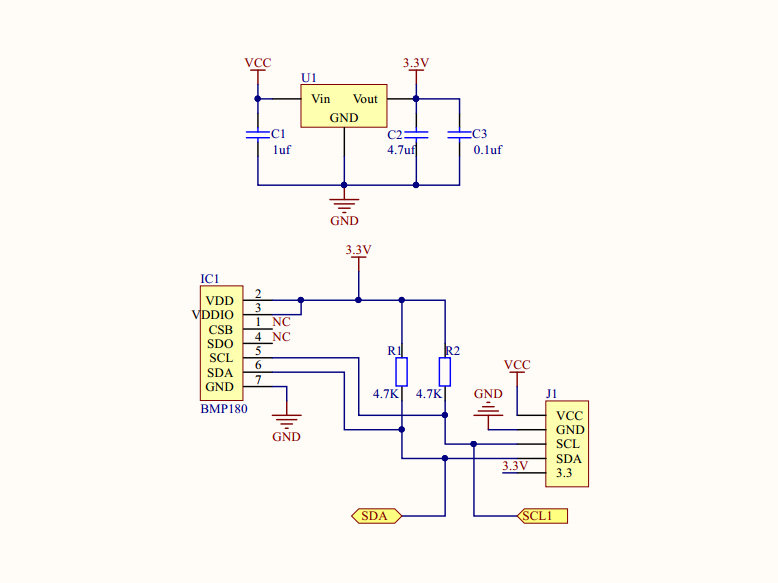Hardware Setup
Assembling the Circuit as followed Connection GraphSoftware

To use the I2C interface, we should enable the I2C first. Please enter the following command:

sudo  nano  /boot/config.txt

Open the file /boot/config.txt, find the code line”dtparam=i2c_arm”，checking if there is # sign in front of the line, uncomment it (remove the # in front of this line), and make sure the end of the line is”on”, finally the code should look like this: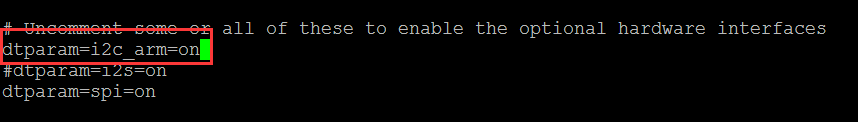Press Ctrl+X，and type “Y” to save the file you revised. reboot Pi.

We’ll provide two kinds of codes for C language users and Python language users.

Please be sure installation wiringpi. If you have not installed wiringPi, read  lesson 3: installation wiringpi

cd ~
wget  http://osoyoo.com/driver/pi3_start_learning_kit_lesson_18/bmp180-c.tar.gz
sudo   tar  zxvf   bmp180-c.tar.gz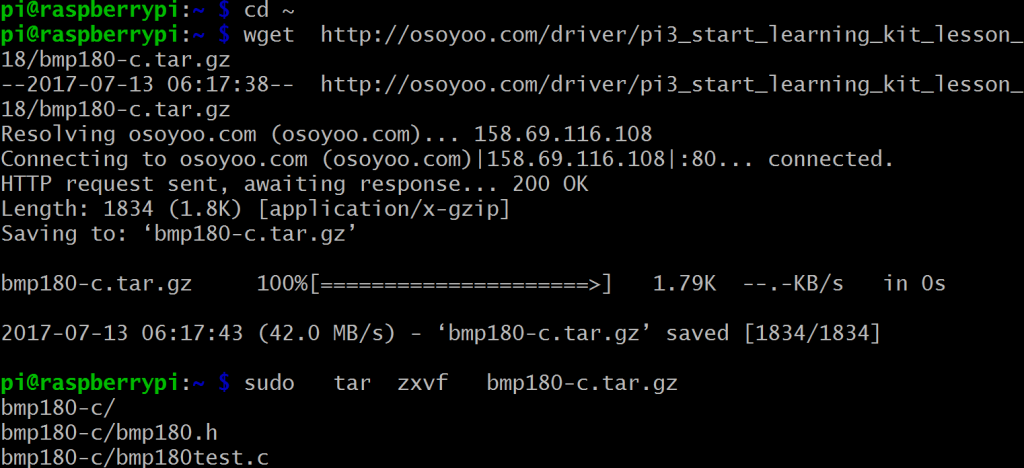Step 2) Change the path
cd   bmp180-c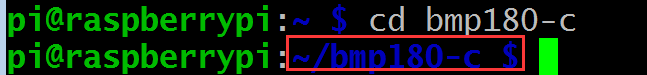Step 3) Compile code
sudo gcc -Wall -o bmp180 bmp180test.c -lwiringPi -lm

Step 4) Run program
sudo  ./bmp180
Program result
Once the program starts running , the terminal will show pressure, temperature and altitude which BMP180 sensor detects.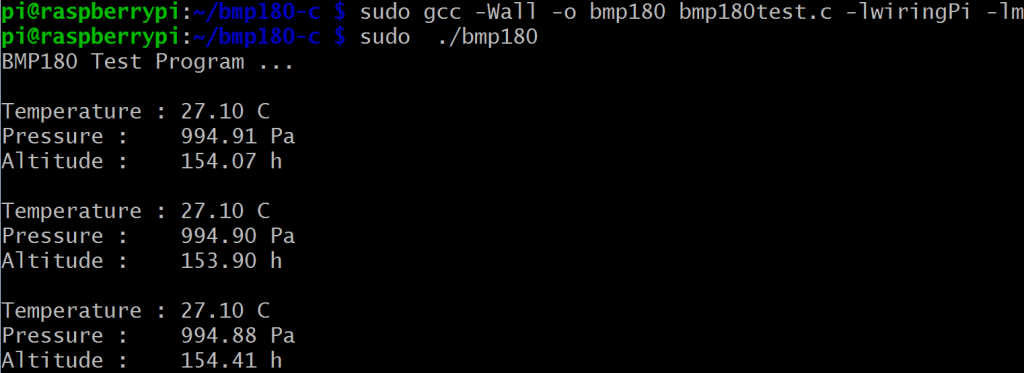bmp180.h and bmp180test.c Code

```#ifndef _BMP180_
#define _BMP180_

//Operating Modes
#define BMP180_ULTRALOWPOWER    0
#define BMP180_STANDARD         1
#define BMP180_HIGHRES          2
#define BMP180_ULTRAHIGHRES     3

//BMP185 Registers
#define BMP180_CAL_AC1          0xAA  //Calibration data (16 bits)
#define BMP180_CAL_AC2          0xAC  //Calibration data (16 bits)
#define BMP180_CAL_AC3          0xAE  //Calibration data (16 bits)
#define BMP180_CAL_AC4          0xB0  //Calibration data (16 bits)
#define BMP180_CAL_AC5          0xB2  //Calibration data (16 bits)
#define BMP180_CAL_AC6          0xB4  //Calibration data (16 bits)
#define BMP180_CAL_B1           0xB6  //Calibration data (16 bits)
#define BMP180_CAL_B2           0xB8  //Calibration data (16 bits)
#define BMP180_CAL_MB           0xBA  //Calibration data (16 bits)
#define BMP180_CAL_MC           0xBC  //Calibration data (16 bits)
#define BMP180_CAL_MD           0xBE  //Calibration data (16 bits)
#define BMP180_CONTROL          0xF4
#define BMP180_TEMPDATA         0xF6
#define BMP180_PRESSUREDATA     0xF6

//Commands

#endif```
```#include < wiringPi.h>
#include < wiringPiI2C.h>
#include < stdio.h>
#include < math.h>
#include "bmp180.h"

#define OSS BMP180_STANDARD
short AC1,AC2,AC3,B1,B2,MB,MC,MD;
unsigned short AC4,AC5,AC6;
int fd;
{
}

{
int MSB,LSB;
int value = (MSB << 8) +LSB; return (unsigned short)value; } short I2C_readS16(int reg) { int result; result = I2C_readU16(reg); if (result > 32767)result -= 65536;
return (short)result;
}
void I2C_writeByte(int reg,int val)
{
wiringPiI2CWriteReg8(fd,reg,val);
}

{
}
{
int raw;
delay(5);  //5ms;
return raw;

}
{
int MSB,LSB,XLSB,raw;
switch(OSS)
{
case BMP180_ULTRALOWPOWER:
delay(5);break;
case BMP180_HIGHRES:
delay(14);break;
case BMP180_ULTRAHIGHRES:
delay(26);break;
default :
delay(8);
}
raw = ((MSB << 16) + (LSB << 8) + XLSB) >> (8 - OSS);
return raw;
}
{
float T;
int UT,X1,X2,B5;
X1 = ((UT - AC6)*AC5) >> 15;
X2 = (MC << 11) / (X1 + MD); B5 = X1 + X2; T = ((B5 + 8) >> 4) /10.0;
return T;
}

{
int P;
int UT,UP,X1,X2,X3,B3,B5,B6;
unsigned int B4;
int B7;

X1 = ((UT - AC6)*AC5) >> 15;
X2 = (MC << 11) / (X1 + MD); B5 = X1 + X2; //Pressure Calculations B6 = B5 - 4000; X1 = (B2 * (B6 * B6) >> 12) >> 11;
X2 = (AC2 * B6) >> 11;
X3 = X1 + X2;
B3 = (((AC1 * 4 + X3) << OSS) + 2) / 4; X1 = (AC3 * B6) >> 13;
X2 = (B1 * ((B6 * B6) >> 12)) >> 16;
X3 = ((X1 + X2) + 2) >> 2;
B4 = (AC4 * (X3 + 32768)) >> 15;
B7 = (UP - B3) * (50000 >> OSS);
if (B7 < 0x80000000){P = (B7 * 2) / B4;} else {P = (B7 / B4) * 2;} X1 = (P >> 8) * (P >> 8);
X1 = (X1 * 3038) >> 16;
X2 = (-7357 * P) >> 16;
P = P + ((X1 + X2 + 3791) >> 4);
return P;

}
{
float pressure,altitude;
float sealevel_pa = 101325.0;
altitude = 44330.0 * (1.0 - pow(pressure / sealevel_pa,(1.0/5.255)));
return altitude;
}
{
float altitude_m = 0.0;
float pressure,p0;
p0 = pressure / pow(1.0 - altitude_m/44330.0,5.255);
return p0;
}
int main(int argc,char **argv)
{
printf("BMP180 Test Program ...\n");
if(wiringPiSetup() < 0) return 1;
while(1)
{
delay(1000);
}
return 0;
```

For python users

1) Please install a python tool software (smbus) to communicate with IIC

cd  ~

sudo apt-get install python-smbus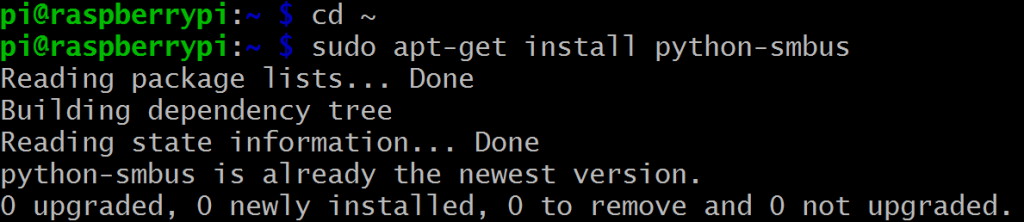cd  ~

wget  http://osoyoo.com/driver/pi3_start_learning_kit_lesson_18/bmp180-python.tar.gz

sudo  tar zxvf  bmp180-python.tar.gz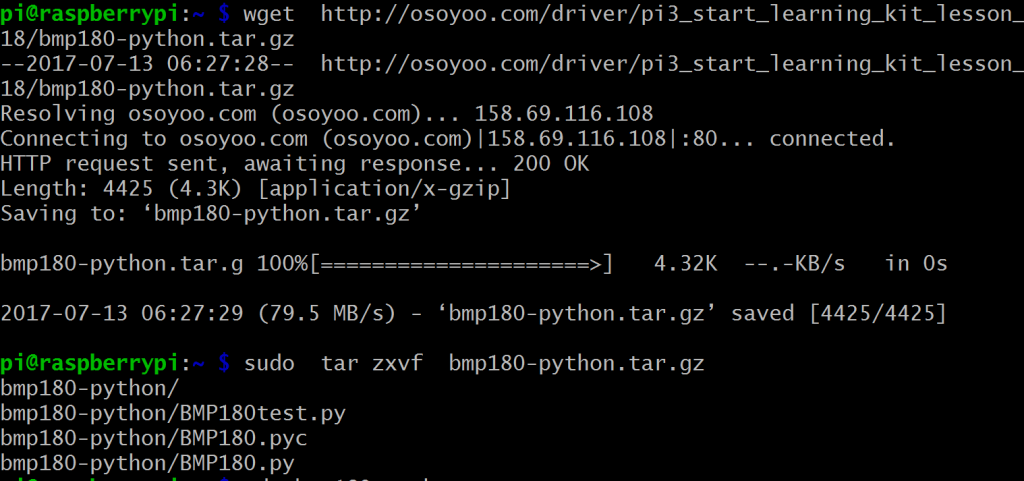3) Change the path

cd  bmp180-python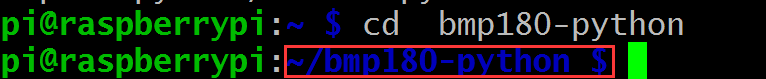4) Run program

sudo  python  ./BMP180test.py

5) Program result

Once the program starts running , the terminal will show pressure, temperature and altitude which BMP180 sensor detects.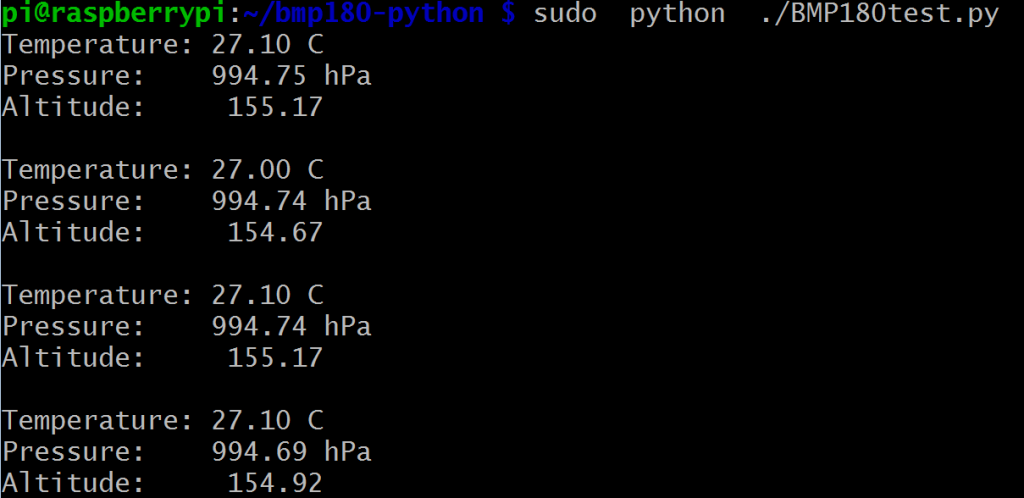BMP180.py, BMP180.pyc and BMP180test.py code

```import time
import smbus

# Operating Modes
BMP180_ULTRALOWPOWER     = 0
BMP180_STANDARD          = 1
BMP180_HIGHRES           = 2
BMP180_ULTRAHIGHRES      = 3

# BMP085 Registers
BMP180_CAL_AC1           = 0xAA  # R   Calibration data (16 bits)
BMP180_CAL_AC2           = 0xAC  # R   Calibration data (16 bits)
BMP180_CAL_AC3           = 0xAE  # R   Calibration data (16 bits)
BMP180_CAL_AC4           = 0xB0  # R   Calibration data (16 bits)
BMP180_CAL_AC5           = 0xB2  # R   Calibration data (16 bits)
BMP180_CAL_AC6           = 0xB4  # R   Calibration data (16 bits)
BMP180_CAL_B1            = 0xB6  # R   Calibration data (16 bits)
BMP180_CAL_B2            = 0xB8  # R   Calibration data (16 bits)
BMP180_CAL_MB            = 0xBA  # R   Calibration data (16 bits)
BMP180_CAL_MC            = 0xBC  # R   Calibration data (16 bits)
BMP180_CAL_MD            = 0xBE  # R   Calibration data (16 bits)
BMP180_CONTROL           = 0xF4
BMP180_TEMPDATA          = 0xF6
BMP180_PRESSUREDATA      = 0xF6

# Commands

class BMP180(object):
self._mode = mode
self._bus = smbus.SMBus(1)

return (MSB << 8) + LSB def _read_s16(self,cmd): result = self._read_u16(cmd) if result > 32767:result -= 65536
return result

def _write_byte(self,cmd,val):

"""Reads the raw (uncompensated) temperature from the sensor."""
time.sleep(0.005)  # Wait 5ms
raw = (MSB << 8) + LSB
return raw

"""Reads the raw (uncompensated) pressure level from the sensor."""
self._write_byte(BMP180_CONTROL, BMP180_READPRESSURECMD + (self._mode << 6))
if self._mode == BMP180_ULTRALOWPOWER:
time.sleep(0.005)
elif self._mode == BMP180_HIGHRES:
time.sleep(0.014)
elif self._mode == BMP180_ULTRAHIGHRES:
time.sleep(0.026)
else:
time.sleep(0.008)
raw = ((MSB << 16) + (LSB << 8) + XLSB) >> (8 - self._mode)
return raw

"""Gets the compensated temperature in degrees celsius."""

X1 = ((UT - self.cal_AC6) * self.cal_AC5) >> 15
X2 = (self.cal_MC << 11) / (X1 + self.cal_MD) B5 = X1 + X2 temp = ((B5 + 8) >> 4) / 10.0
return temp

"""Gets the compensated pressure in Pascals."""

X1 = ((UT - self.cal_AC6) * self.cal_AC5) >> 15
X2 = (self.cal_MC << 11) / (X1 + self.cal_MD) B5 = X1 + X2 # Pressure Calculations B6 = B5 - 4000 X1 = (self.cal_B2 * (B6 * B6) >> 12) >> 11
X2 = (self.cal_AC2 * B6) >> 11
X3 = X1 + X2
B3 = (((self.cal_AC1 * 4 + X3) << self._mode) + 2) / 4 X1 = (self.cal_AC3 * B6) >> 13
X2 = (self.cal_B1 * ((B6 * B6) >> 12)) >> 16
X3 = ((X1 + X2) + 2) >> 2
B4 = (self.cal_AC4 * (X3 + 32768)) >> 15
B7 = (UP - B3) * (50000 >> self._mode)

if B7 < 0x80000000: p = (B7 * 2) / B4 else: p = (B7 / B4) * 2 X1 = (p >> 8) * (p >> 8)
X1 = (X1 * 3038) >> 16
X2 = (-7357 * p) >> 16

p = p + ((X1 + X2 + 3791) >> 4)
return p

"""Calculates the altitude in meters."""
# Calculation taken straight from section 3.6 of the datasheet.
altitude = 44330.0 * (1.0 - pow(pressure / sealevel_pa, (1.0/5.255)))
return altitude

"""Calculates the pressure at sealevel when given a known altitude in
meters. Returns a value in Pascals."""
p0 = pressure / pow(1.0 - altitude_m/44330.0, 5.255)
return p0```
```import time
from BMP180 import BMP180

# Initialise the BMP085 and use STANDARD mode (default value)
# bmp = BMP085(0x77, debug=True)
bmp = BMP180()

# To specify a different operating mode, uncomment one of the following:
# bmp = BMP085(0x77, 0)  # ULTRALOWPOWER Mode
# bmp = BMP085(0x77, 1)  # STANDARD Mode
# bmp = BMP085(0x77, 2)  # HIRES Mode
# bmp = BMP085(0x77, 3)  # ULTRAHIRES Mode
while True:

# Read the current barometric pressure level

# To calculate altitude based on an estimated mean sea level pressure
# (1013.25 hPa) call the function as follows, but this won't be very accurate